The internal resistance of a 2.1V cell which gives a current of 0.2A through a resistance of 10Ω is :

(a)0.2Ω

(b)0.5Ω

(c)0.8Ω

(d)1.0Ω

Concept Questions :-

Emf and terminal voltage
High Yielding Test Series + Question Bank - NEET 2020

Difficulty Level:

A millivoltmeter of 25 mV range is to be converted into an ammeter of 25 A range. The value (in ohm) of necessary shunt will be

(a)0.001                                     (b)0.01

(c)1                                            (d)0.05

High Yielding Test Series + Question Bank - NEET 2020

Difficulty Level:

In the circuit shown the cells A and B have negligible resistances. For  the galvanometer (g) shown no deflection.The value of ${V}_{B}$ is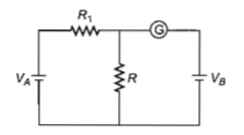(a)4V                                         (b)2V

(c)12V                                       (d)6V

High Yielding Test Series + Question Bank - NEET 2020

Difficulty Level:

If voltage across a bulb rated 220 V-100 W drops by 2.5% of its rated value, the percentage of the rated value by which the power would decrease is

(a)20%                                             (b)2.5%

(c)5%                                               (d)10%

High Yielding Test Series + Question Bank - NEET 2020

Difficulty Level:

A ring is made of a wire having a resistane

${\mathrm{R}}_{0}=12\mathrm{\Omega }$. Find the points A and B, as shown

in the figure, at which a current carrying

conductor should be connected so that the

resistance R of the sub circuit between these

points is equal to 8/3$\mathrm{\Omega }$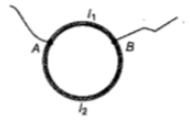1. $\frac{{\mathrm{l}}_{1}}{{\mathrm{l}}_{2}}=\frac{5}{8}$                    2. $\frac{{\mathrm{l}}_{1}}{{\mathrm{l}}_{2}}=\frac{1}{3}$

3. $\frac{{\mathrm{l}}_{1}}{{\mathrm{l}}_{2}}=\frac{3}{8}$                    4. $\frac{{\mathrm{l}}_{1}}{{\mathrm{l}}_{2}}=\frac{1}{2}$

Concept Questions :-

Combination of resistors
High Yielding Test Series + Question Bank - NEET 2020

Difficulty Level:

The power dissipated in the circuit shown in

the figure is 30 Watt. The value of R is :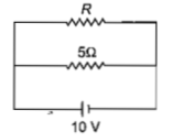(a) $20\mathrm{\Omega }$

(b) $15\mathrm{\Omega }$

(c) $10\mathrm{\Omega }$

(d) $30\mathrm{\Omega }$

Concept Questions :-

Heating effect of current
High Yielding Test Series + Question Bank - NEET 2020

Difficulty Level:

A cell having an emf $\mathrm{\epsilon }$ internal resistance r

is connected across a variable external

resistance R. As the resistance R is increased,

the plot of potential difference V across R is given

by

(a)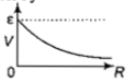(b)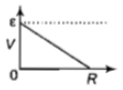(c)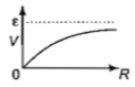(d)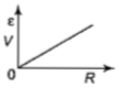Concept Questions :-

Emf and terminal voltage
High Yielding Test Series + Question Bank - NEET 2020

Difficulty Level:

If power dissipated in the 9$\mathrm{\Omega }$ resistor in the circuit

shown is 36 W, the potential difference across

the 2$\mathrm{\Omega }$ resistor is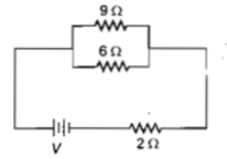(a) 8 V

(b) 10 V

(c) 2 V

(d) 4 V

Concept Questions :-

Heating effect of current
High Yielding Test Series + Question Bank - NEET 2020

Difficulty Level:

A current of 2A flows through a 2$\mathrm{\Omega }$ resistor

when connected across a battery. The same

battery supplies a current of 0.5 A when

connected across a 9$\mathrm{\Omega }$ resistor. The internal

resistance of the battery is

(a) $1/3\mathrm{\Omega }$                   (b) $1/4\mathrm{\Omega }$

(c) $1\mathrm{\Omega }$                       (d) $0.5\mathrm{\Omega }$

Concept Questions :-

Emf and terminal voltage
High Yielding Test Series + Question Bank - NEET 2020

Difficulty Level:

In the circuit in the figure, if the potential at point A is taken to be zero, the potential at point B is :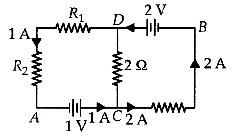1. -1V                                          2. +2V

3. -2V                                          4. +1V

Concept Questions :-

Kirchoff's voltage law